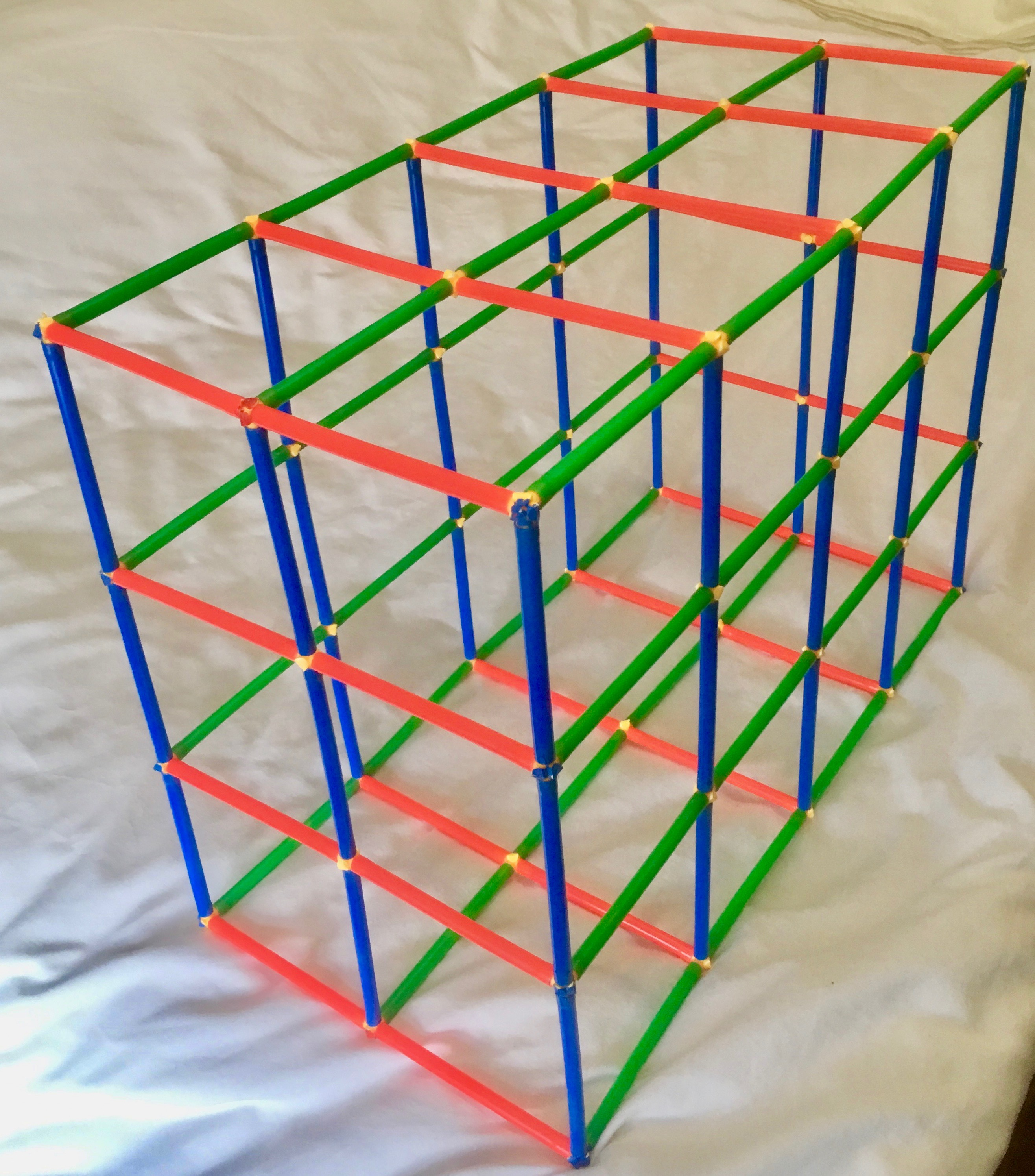# Wire Cages

## Wire Cages

#### Wire cages are used to contain large rocks in some building projects.

Here is a small version of a 2 by 3 by 4 wire cage cuboid, made with plastic straws.We can see 14 red rods of length 2 units, 12 blue rods of length 3 units and 10 green rods of length 4 units.

### Challenge 1

The example above of dimensions 2 by 3 by 4 uses exactly 36 rods and has a volume of 24 cube units.

Find all the cages that have a volume of 20 to 25 cube units inclusive and use 40 to 50 rods inclusive.

### Challenge 2

The example above of dimensions 2 by 3 by 4 uses exactly 36 rods.

Can you find a cuboid that uses exactly 28 rods?
Can you find all the cuboids that use exactly 28 rods?

### Challenge 3

Find at least one pair of cuboids of different dimensions, that when constructed:
- Use exactly 100 rods
AND
- Have each dimension greater than 2 rods.

### Why do this problem?

This task was designed for the 2018 National Young Mathematicians' Award, so it might be a particularly useful activity for a small group of your highest-attaining pupils to work on. It encourages a systematic approach and provides an opportunity for pupils to explain how they know they have all the solutions.

### Possible approach

Begin by showing a model 'wire cage' like the one in the picture. Discuss how many long rods are used to make it, and what the lengths of these rods are. How can we find the volume of a cuboid? Make sure that pupils understand that the volume is the number of small cubes (one unit long in each direction) that can fit inside their cuboid - this will help them visualise how the volume changes when the lengths change.

There is a great interactive resource here (in the 'Getting Started' section) which can be used to explore different cuboids.

When pupils understand the key ideas about 'wire cages', give them time to work in small groups on the three challenges.

### Key questions

Explain what you are doing to find the next solution.
Tell me about the number of rods that you have.
How do you know what the volume of that cuboid is?
How are you trying to get a particular total?

### Possible extension

Pupils could be encouraged to consider what happens when you start with a small cuboid and increase one of the dimensions by 1 many times, e.g. 1x2x3, 2x2x3, 3x2x3, 4x2x3... Then they could think about how many rods are needed for each and what the relationship is between the numbers.

### Possible support

Some pupils may need some model making materials or pictures of other cuboids to help them visualise their 'wire cages'.
Sharing different ways of thinking about the problem and different ways of recording that thinking can be very useful in developing all learners' problem-solving skills.